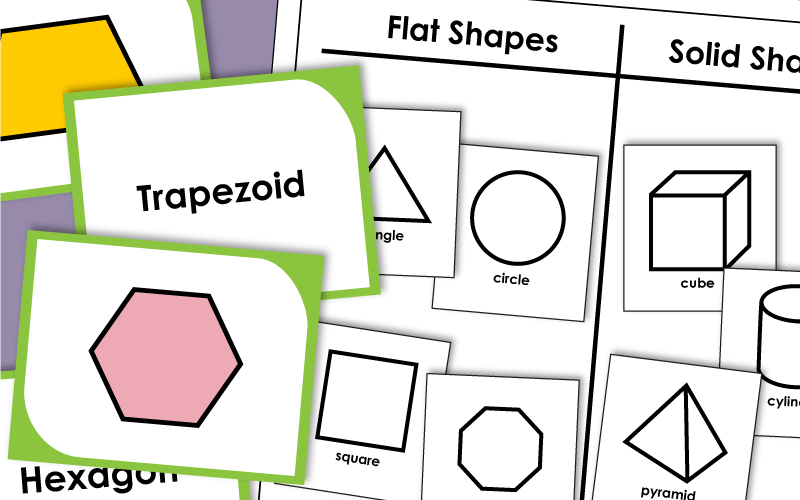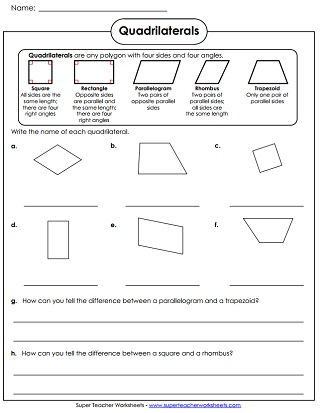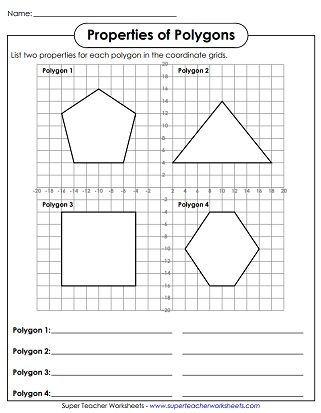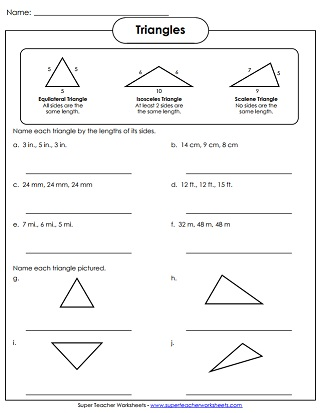# Polygon Worksheets : Flat Shapes

This page contains printable polygon worksheets.  Flat shapes (plane figures) include triangles, quadrilaterals (squares, rectangles, rhombuses, parallelograms), pentagons, hexagons, and octagons.## Polygons

Identify each shape.  Includes solid figures (3D shapes) and polygons (flat shapes).
Mystery picture creates a triangle, quadrilateral, pentagon, hexagon, and octagon.  After revealing the mystery picture, students must color each polygon shape according to the instructions.
In this card game, players match up the polygon or solid shapes with their names.
Determine the number of sides, parallel lines, and right angles are in each polygon shape.
List two properties for each of the four polygons shown on the coordinate plane.
Color, cut, and fold the origami fortune teller (a.k.a. cootie catcher). Then play the game to review the names of basic polygon shapes.
Use Microsoft Paint to create a polygon picture.  Label the sides and angles on each polygon.
Make flat shapes using toothpicks and clay. Keep track of the shapes build on the chart.

Name the quadrilaterals shown: rhombus, parallelogram, square, rectangle, or trapezoid
Match the definition on the left with the quadrilateral type on the right.
Cut out the eight shapes at the bottom. Glue them into the correct positions on the chart. Sort by angles and polygon type (quadrilaterals).

## Triangles

Identify the type of triangles shown: equilateral, isosceles, and scalene triangles.
Identify the type of triangles shown: acute, obtuse, and right triangles.

## Flat and Solid Shaped (Mix)

Cut out the shapes and glue them onto the t-chart. There is a column for flat shapes, and a column for solid shapes.

Geometry Worksheets

Worksheets for teaching coordinate grids, ordered pairs, and plotting points.

Solid Shapes

Geometry worksheets for solid (3D) shapes

Similar and Congruent Shapes

Printable on identifying similar and congruent shapes

Symmetry Worksheets

Several symmetry activities and printable worksheets

## Worksheet ImagesMy Account
Site Information